## Singular Point (Differential Equation)

Consider a second-order Ordinary Differential EquationIfandremain Finite at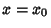, then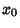is called an Ordinary Point. If eitherordiverges as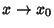, thenis called a singular point. Singular points are further classified as follows:
1. If eitherordiverges asbutand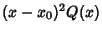remain Finite as, thenis called a Regular Singular Point (or Nonessential Singularity).

2. Ifdiverges more quickly than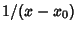, soapproaches Infinity as, ordiverges more quickly than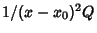so thatgoes to Infinity as, thenis called an Irregular Singularity (or Essential Singularity).

Arfken, G. Singular Points.'' §8.4 in Mathematical Methods for Physicists, 3rd ed. Orlando, FL: Academic Press, pp. 451-454, 1985.